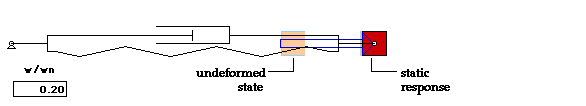# Forced response - harmonic excitation

Consider an undamped single degree-of-freedom system experiencing harmonic excitation whose equation of motion (EOM) is given by:Here omegan is known as the natural frequency of response.

The particular solution for this EOM is give by:where the magnitude of  A is known as the "amplitude of response". A plot of A vs. omega the frequency of excitation omega is shown below.Observations on A as a function of excitation frequency

For low frequencies of excitation (omega < omegan ), the response amplitude A is approximately F0/K, or the static response for a constant load of F0, as shown in the animation below. It is also seen that the response is "in phase" with the excitation (since A > 0).

 Frequency of excitation near zero The response is in phase with the excitation and the amplitude of response approaches the static response.For near-resonant  frequencies of excitation (omega approximately equal to omegan), the magnitude of  A is large, as shown in the animation below. It can be shown that the response is "90 degrees out of phase" with the excitation.

Frequency of excitation near resonance
The response is nearly 90 degrees out of phase with the excitation and the amplitude of response is large.For high frequencies of excitation (omega > omegan ), the magnitrude of the response amplitude A is very small, as shown in the animation below. It is also seen that the response is "180 degrees out of phase" with the excitation (since A < 0).

 Large frequency of excitation The response is 180 degrees out of phase with the excitation and the amplitude of response is small.